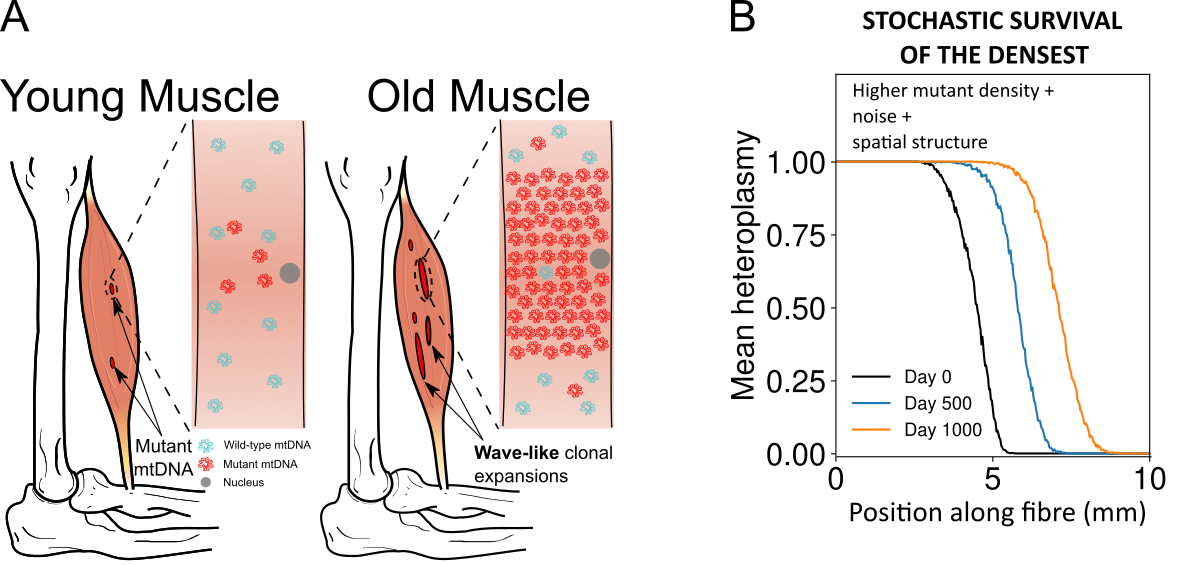## Tuesday 8 August 2017

### What we learn from the learning rate

Cells need to sense their environment in order to survive. For example, some cells measure the concentration of food or the presence of signalling molecules. We are interested in studying the physical limits to sensing with limited resources, to understand the challenges faced by cells and to design synthetic sensors.

We have recently published a paper 'What we learn from the learning rate' (free version) where we explore the interpretation of a metric called 'the learning rate' that has been used to measure the quality of a sensor (e.g here). Our motivation is that in this field a number of metrics (a metric is a number you can calculate from the properties of the sensor that, ideally, tells you how good the sensor is) have been applied to make some statement about the quality of sensing, or limits to sensory performance. For example, a limit of particular interest is the energy required for sensing. However, it is not always clear how to interpret these metrics. We want to find out what the learning rate means. If one sensor has a higher learning rate than another what does that tell you?

The learning rate is defined as the rate at which changes in the sensor increase the information the sensor has about the signal. The information the sensor has about the signal is how much your uncertainty about the state of the signal is reduced by knowing the state of the sensor (this is known as the mutual information). From this definition, it seems plausible that the learning rate could be a measure of sensing quality, but it is not clear. Our approach is a test to destruction – challenge the learning rate in a variety of circumstances, and try to understand how it behaves and why.

To do this we need a framework to model a general signal and sensor system. The signal hops between discrete states and the sensor also hops between discrete states in a way that follows the signal. A simple example is a cell using a surface receptor to detect the concentration of a molecule in its environment.

The figure shows such a system. The circles represent the states and the arrows represent transitions between the states. The signal is the concentration of a molecule in the cell’s environment. It can be in two states; high or low, where high is double the concentration of low. The sensor is a single cell surface receptor, which can be either unbound or bound to a molecule. Therefore, the joint system can be in four different states. The concentration jumps between its states with rates that don’t depend on the state of the sensor. The receptor becomes unbound at a constant rate and is bound at a rate proportional to the molecule concentration.

We calculated the learning rate for several systems, including the one above, and compared it to the mutual information between the signal and the sensor (the is a refined measure of correlation). We found that in the simplest case, shown in the figure, the learning rate essentially reports the correlation between the sensor and the signal and so it is showing you the same thing as the mutual information. In more complicated systems the learning rate and mutual information show qualitatively different behaviour. This is because the learning rate actually reflects the rate at which the sensor must change in response to the signal, which is not, in general, the equivalent to the strength of correlations between the signal and sensor. Therefore, we do not think that the learning rate is useful as a general metric for the quality of a sensor. Rory, Nick and Tom

### Stochastic Survival of the Densest: defective mitochondria could be seen as altruistic to understand their expansion

With age, our skeletal muscles (e.g. muscle of our legs and arms) work less well. In some people, there is a substantial loss of strength an...